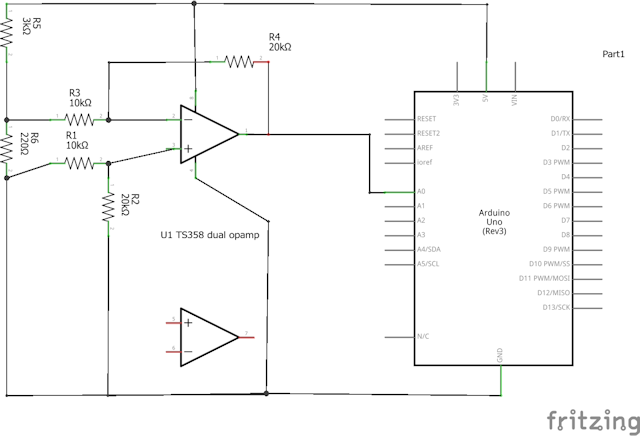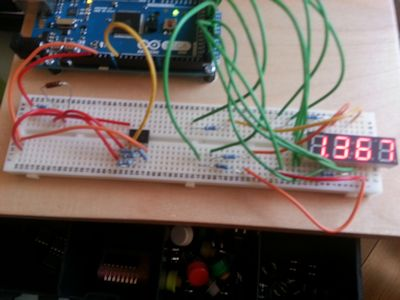By dave | April 27, 2014

I’ve got a side project of building a digitally Arduino controlled PSU that also includes an accurate component tester. In order to do this I’ll have to be able to monitor the current flowing through a circuit, so I’d need some way of monitoring this. Before continuing, I’d like to say that is not an exemplar of how to build a great current sensing circuit, it’s a prototype shown for example purposes only.

In order to measure current, we need to measure the difference in voltage across a resistor. In this case I am using an operational amplifier (opamp TS358) for the job and it is using R6 for this measurement. For this example I’ve chosen a very large value of current sensing resistor as this is a proof of concept and it makes testing easier. Calculating current is a simple case of Ohms law based on the voltage across the opamps + and - inputs and the resistor R6:

``````I=V(r6) / R(r6)

therefore:

I = V(opampOutput) / 220
``````If we then combine this with the LEDDisplay library and a multisegment 7segment display we can output the current through the resistor on the display. To do this we need to know the opamp as approximately 2x gain (Av = R4/R3), and that 1mA across R6 creates about 220mV (which multiplied by the gain of 2 becomes about 440mV). Make sure you’ve set up the 7 segment display as per the instructions in the link above and then run the following code in Arduino Studio.

``````#include <LEDDisplay.h>
void setup()
{
// LED connections start at output 32,
// common must be HIGH to be on.
LEDDisplay* d = new LEDDisplay(32, true);
d->startInterrupt();
}
void loop()
{
// we need to divide by 220 in order for this to show the current.
float d = (float)analogRead(0) / (1024.0 / 220.0) ;
LEDDisplay::instance()->setValueFloat(d, 3);
delay(50);
}

``````

Lastly here it is running on my Arduino prototyping board with pretty much the exact circuit above and the 7segment display wired up.